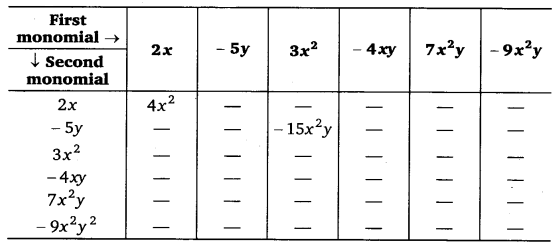# Class 8 Maths NCERT Solutions for Chapter – 9 Algebraic Expressions and Identities Ex – 9.2

### Algebraic Expressions and Identities

Question 1.
Find the product of the following pairs of monomials :
(i)
4, 7p
(ii) -4p, 7p
(iii) – 4p, 7pq
(iv) 4p3 , – 3p
(v) 4p, 0

Solution:
(i) 4 x 7p = (4 x 7) x p = 28p
(ii) – 4 p x 7p = (- 4 x 7) x (px P)
= -28p1 + 1 = – 28p2
(iii) -4px 7pq = (- 4 x 7) x (p x p x q)
= -28 p1+1q = – 28p2q
(iv) 4p3 x – 3p = (4 x – 3) x (p3 x p)
= – 12p3+1 = =-12p4
(v) 4p x 0 = (4 x 0) x p = 0 x p = 0

Question 2.
Find the areas of rectangles with the following pairs of monomials as their lengths and breadths respectively :
(p, q); (10m, 5n); (20x2, 5y2); (4x, 3x2); (3mn, 4np)

Solution:
We know that the area of a rectangle = l x b, where l = length and b = breadth.
Therefore, the areas of rectangles with pair of monomials (p, q); (10m, 5n); (20x2, 5y2); (4x, 3x2) and (3mn, 4np) as their lengths and breadths are given by
pxq=pq
10 m x 5n = (10 x 5) x (m x n) = 50 mn
20x2 x 5y2 = (20 x 5) x (x2 x y2) = 100x2y2
4x x 3x2 = (4 x 3) x (x x x2)
= 12x2
and, 3 mn x 4np = (3×4 )x(mxnxnxp)
=12 mn2p

Question 3.
Complete the table of products :Solution:
Completed table is as under :Question 4.
Obtain the volume of rectangular boxes with the following length, breadth and height respectively
(i)
5a, 3a2, 7a4
(ii) 2p, 4q, 8r
(iii) xy, 2x2y, 2xy2
(iv) a, 2b, 3c

Solution:
(i) Required volume = 5a x 3a2 x 7a4
= (5 x 3 x 7) x (a x a2 x a4)
= 105a1+2+4 =105a7

(ii) Required volume = 2p x 4q x 8r
= (2 x 4 x 8 )x p x q x r = 64 pqr

(iii) Required volume =xy x 2x2y x 2xy2
= (1 x 2 x 2) x (x x x2 x x x y x y x y2)
= 4x1+2+1 y1+1+2 = 4x4y4

(iv) Required volume =a x 2b x 3c
= (1 x 2 x 3) x (a x b x c)
= 6abc

Question 5.
Obtain the product of
(i)
xy, yz, zx
(ii) a, – a2, a3
(iii) 2, 4y, 8y2, 16y3
(iv) a, 26, 3c, 6a6c
(v) m, – mn, mnp

Solution: